## Atoms, Molecules, and Ions

Isotopes are atoms of the same element that have the same atomic number but different mass numbers. Now, because the atomic number represents the number of protons, we can also say that isotopes have the same number of protons but a different number of neutrons.

For example, there are two common isotopes of carbon: carbon-12 and carbon-13. In the periodic table, or in general, their notation will look like this: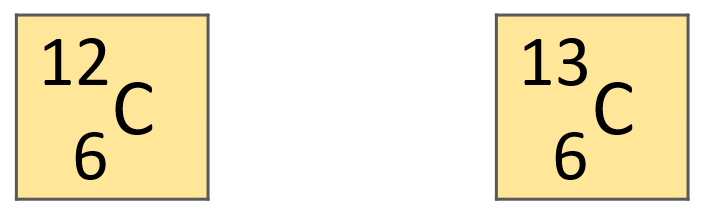The number on the bottom is the atomic number, and the one on the top is the atomic mass or the atomic mass number of the particular isotope.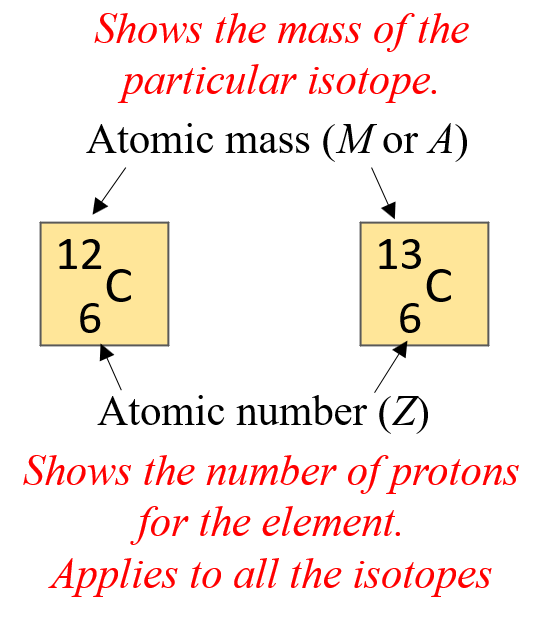You can also remember it this way; the smaller number is the atomic number, and the larger number is the mass number. And the reason for this is, remember, the mass number is the sum of the atomic number (number of protons) and the number of neutrons.

M = atomic number + number of neutrons

M = Z + n

Most often, in the periodic table, you will see a number with decimals instead of a whole number. This is the average atomic mass, which is the average mass of all the isotopes for that element, For example: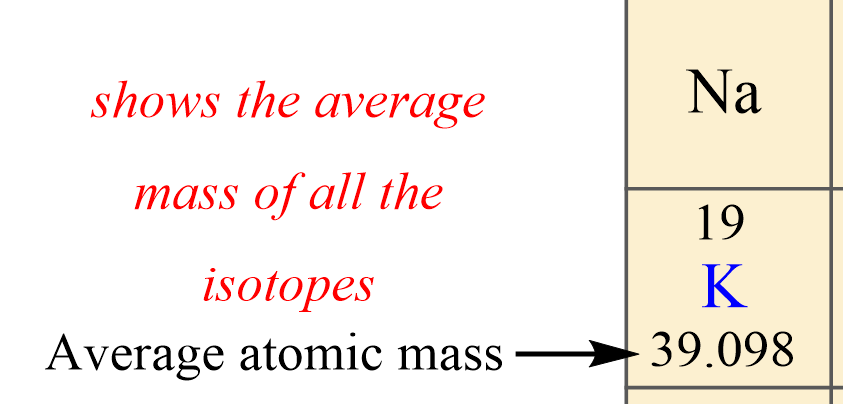So, the top number in this case is the atomic number and the one on the bottom is the average atomic mass K, which is calculated by the weighted average of all the isotopes. That is, the mass of each isotope is multiplied by the percent fraction of its abundance.

# Key Features of Isotopes

The first thing which we have already mentioned is that isotopes have the same atomic number. That is, they have the same number of protons and therefore, the atomic number is like the ID of the element.

Second, remember, that atomic mass is the sum of the number of protons and neutrons. So, if we have one, we can calculate the other.

For example, how many protons does the 37Cl isotope of chlorine have?

What you need to first, is look up the atomic number of Cl. It is 17, so chlorine has 17 protons. Now, to find the number of neutrons, we subtract this number from the atomic mass which is given in the top left corner of the symbol:

# n = 37 – 17 = 20 neutrons

Another important thing you need to remember about the relationship of the particles is that the number of protons in a neutral atom is equal to the number of electrons. This is what makes the atom neutral since the number of positively charged particles is equal to the number of negatively charged particles.

To illustrate this, let’s look at a more descriptive representation of the two carbon isotopes: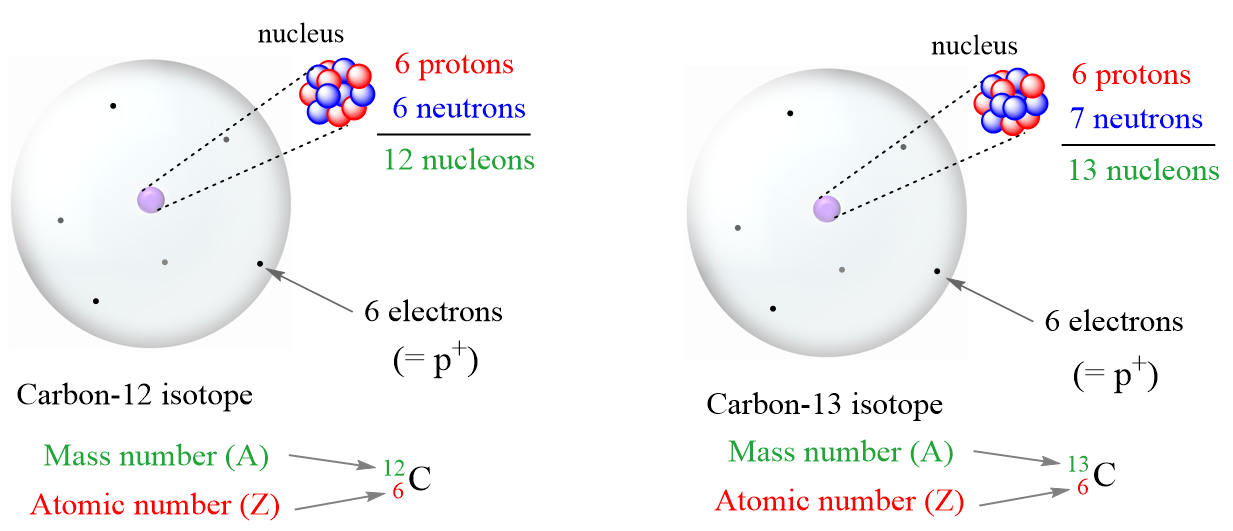It is not the same for ions though because the charge of the ion is a result of an imbalance between the number of protons and electrons. If it is a cation, then the positive indicates how many more protons it has compared to the number of electrons. For anions, the charge tells how many extra electrons there are compared to the number of protons.

Recall, this pattern for the formation of anions and cations:

Metals tend to lose electron(s) and become cations (positively charged ions).Nonmetals tend to gain an electron(s) and become anions (negatively charged ions).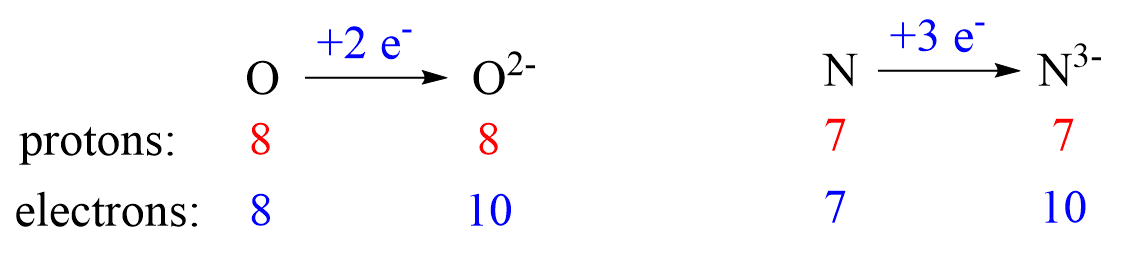Notice that the number of protons is not changed, and the ions are charged because, unlike atoms, their number of protons and electrons is not equal.

# Solving Problems on Isotopes

When working on problems calculating the number of protons, neutrons, and electrons. We are going to need the atomic mass of the specific isotope.

For example, write the symbol of the atom with 15 protons and 16 neutrons.

First, we need a periodic table to look up the element with atomic number 16 which we find is phosphorous, P. Next, we need to find the atomic mass by adding the number of protons and neutrons together:

M = p + n = 15 + 16 = 31

And now we can write the symbol by placing the atomic mass in top left, and the atomic number in the bottom left side of the element:

$_{15}^{31}{\rm{P}}$

You may also be asked to write the atomic symbol based on the number of neutrons.

For example, write the atomic symbol of the element that has a mass number of 107 and 60 neutrons.

This time, we need to determine the number of protons by subtracting the number of neutrons from the mass number:

Z (# p) = M – n = 107 – 60 = 47

Next, we look up the element with the atomic number 47 which is Ag. The atomic symbol for this isotope would be:

$_{47}^{107}{\rm{Ag}}$

In the next article, we will work on examples of collating the number of protons, neutrons, and electrons for atoms and ions given different data.

More practice examples on average atomic mass and isotopes can be found in this multiple-choice quiz:

Check Also